# Doppler’s Effect

When the source of the sound and observer are at rest the frequency of the sound heard by observer which is emitted by source of the sound is not changed. When the source of the sound and observer or both are in motion the frequency of the sound heard by observer which is emitted by source of the sound is changed this is due to the relative motion between the source and observer. Thus the apparent change in frequency of sound wave where there is relative motion between the source and observer is called Doppler’s Effect. This effect is valid only when velocity of sound is greater than velocity of source of sound or velocity of observer.

The different cases of the Doppler’s effect is discussed as follows:-
When the source of the sound is moving towards the observer and the observer is moving towards the source of sound.

Consider source of the sound ‘s’ moving towards the observer with velocity and observer is moving towards the source of sound with velocity vo. If source of sound and observer is at rest and if v be the velocity of sound, then the frequency of sound heard by observer = v/l where be the wavelength of sound emitted by source of sound.
Since, source of sound is moving towards the observer.
Then,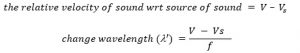Where f is the frequency of sound emitted by source of sound.
Again, the observer is in motion,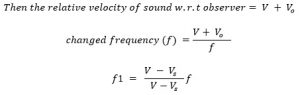This is the changed frequency, when resource of sound is moving towards the observer and observer is moving towards the source of sound. When the observer is moving towards the stationary source of sound. When the observer is moving towards the stationary source of sound with velocity vo. And be the frequency of sound emitted by source of the sound and if the source of the sound and observer is at rest. Then the frequency of sound heard by observer.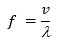Here,
The source of sound is at rest and observer is in motion since the source of sound is rest then the wavelength of sound,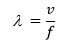But observer is in motion, the relative velocity of sound with respect to the observer.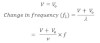This is the apparent frequency when the observer is moving towards the stationary source of s.

Relation between intensity and amplitude of wave.
Suppose the displacement y of the vibration layer of air is given by,
Y=asinωt
Where, A is the amplitude of wave and w is the angular velocity (frequency) of wave
The velocity of vibration layer of air is given by,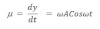And hence corresponding k e is given by,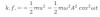Where m is the mass of layer. The layer also has potential energy. And from SHM the total energy of particle executing SHM is maximum KE
i.e. the total energy of vibration air of layer is given by,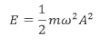In 1sec. air is disturbed by the wave to a distance of v meter where v is the velocity of the sound. If the cross sectional area of the air is taken as then the volume of air disturbed by sound is vm3
M = Sμ
Where S is the density of air.
Then,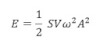This is the energy crossing a unit area per second then by depth of intensity of the sound E is I.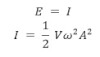i. e. the intensity of the sound of a given frequency is directly proportional to the square of amplitude.